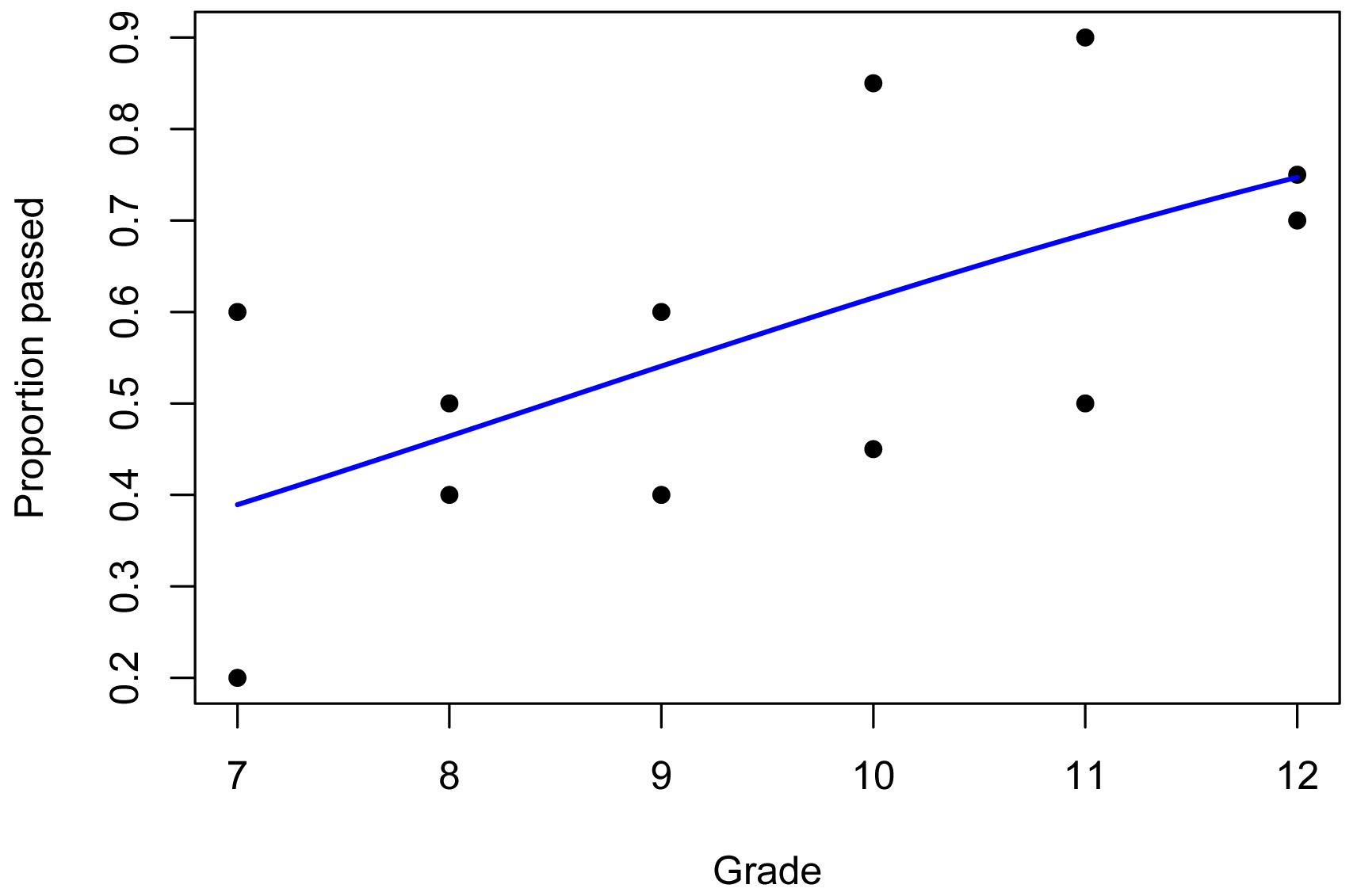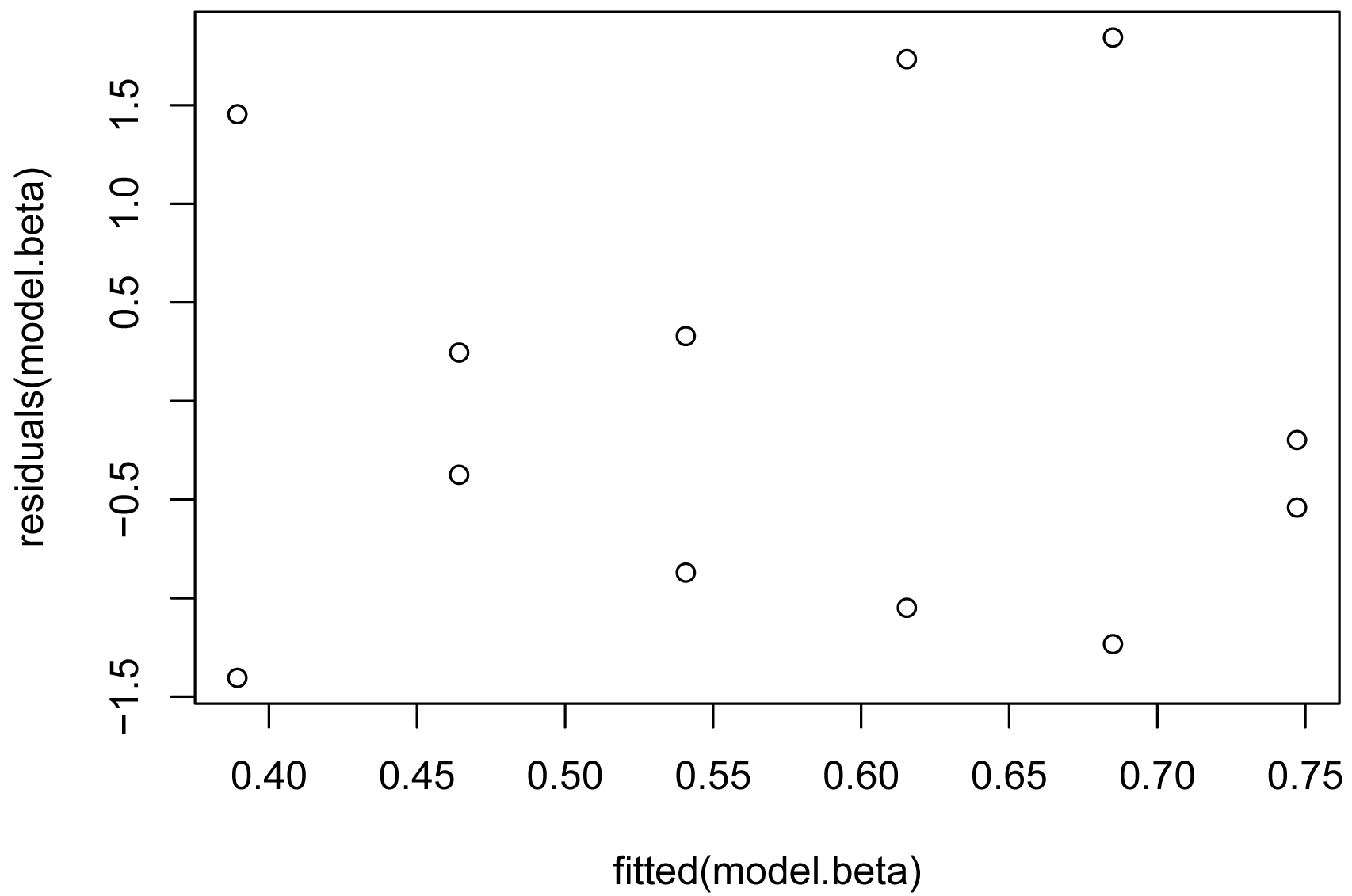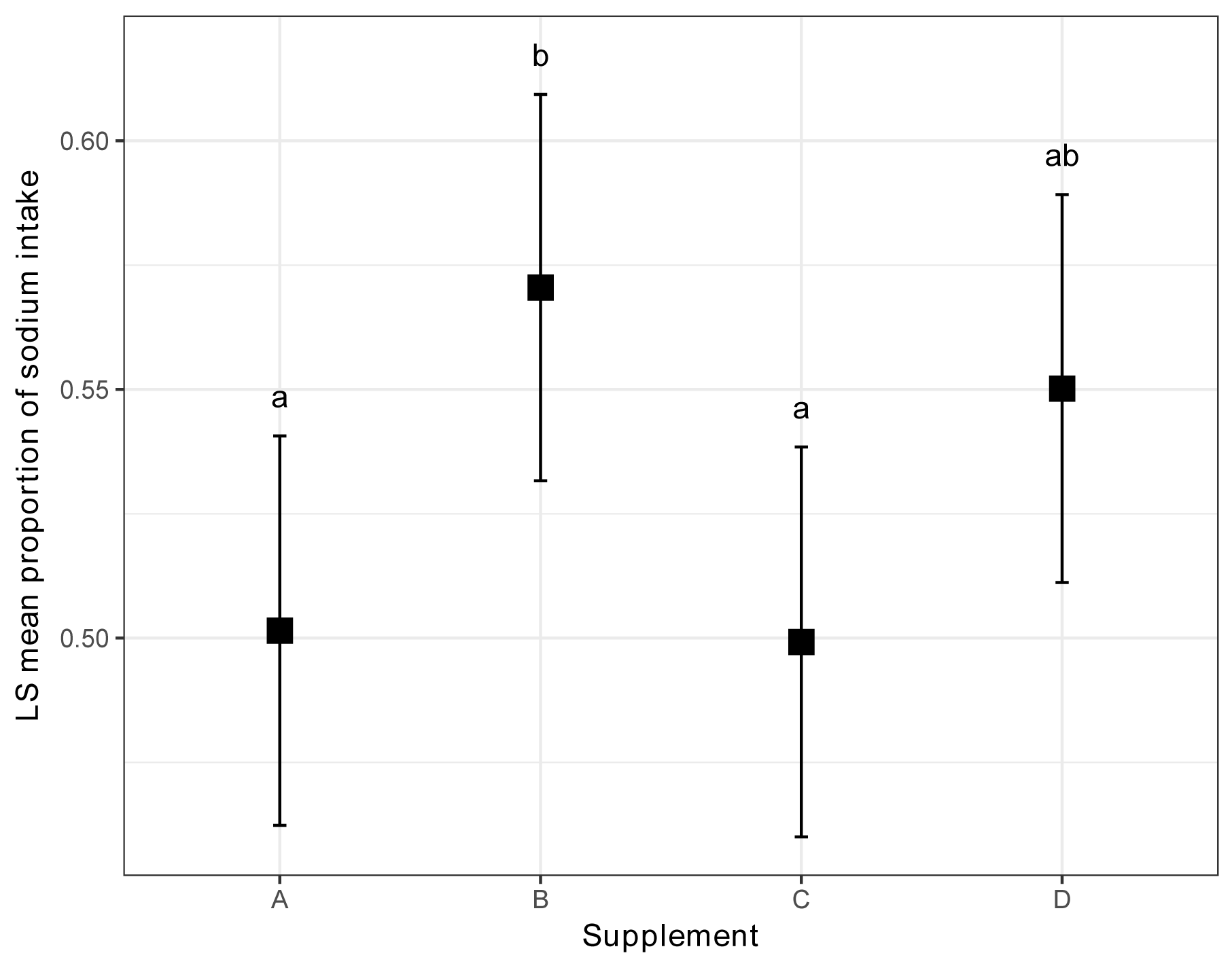## Summary and Analysis of Extension Program Evaluation in R

Salvatore S. Mangiafico

# Beta Regression for Percent and Proportion Data

### Introduction

#### Proportion data

In general, common parametric tests like t-test and anova shouldn’t be used when the dependent variable is proportion data, since proportion data is by its nature bound at 0 and 1, and is often not normally distributed or homoscedastic.

Data of proportions, percentages, and rates can be thought of as falling into a few different categories.

##### Proportion data of discrete counts

Some proportion data is derived from discrete counts of “successes” and “failures”, where the “successes” are divided by the total counts.  The example below with passing and failing counts across classes is an example of this.  Each observation is a percentage from 0 to 100%, or a proportion from 0 to 1.  This kind of data can be analyzed with beta regression or can be analyzed with logistic regression.

##### Proportion data that is inherently proportional

Other proportion data is inherently proportional, in that it’s not possible to count “successes” or “failures”, but instead is derived, for example, by dividing one continuous variable by a given denominator value.  The sodium intake example below is an example of this.  Another case of this kind of proportion data is when a proportion is assessed by subjective measurement.  For example, rating a diseased lawn subjectively on the area dead, such as “this plot is 10% dead, and this plot is 20% dead”.  Each observation is a percentage from 0 to 100%, or a proportion from 0 to 1.  This kind of data can be analyzed with beta regression.

##### Rates with different numerators and denominators

Some rates are expressed with numerators and denominators of different measurements or units.  For example, the number of cases of a disease per 100,000 people or the number of televisions per student’s home.  In these cases, the values are not limited to between 0 and 1, and beta regression is not appropriate.

If the numerator can be considered a count variable, Poisson regression or other methods for count data are usually suggested.  As a complication, often the denominator varies in value.  For example, if the count is disease occurrence in a city and the denominator is the population in a city.  (Each city has a different population.)  Or if the numerator is the count of televisions in the home and the denominator is the number of students in the home.  In these cases, Poisson regression or related methods are often recommended with an offset for the value in the denominator.  These examples are not explored further here, but an example model would be

glm(Count_of_televisions ~ Independent_variable +
offset(log(Number_of_students_in_home)),
family="poisson",
data=Data)

#### Beta regression

Beta regression can be conducted with the betareg function in the betareg package (Cribari-Neto and Zeileis, 2010).  With this function, the dependent variable varies between 0 and 1, but no observation can equal exactly zero or exactly one.  The model assumes that the data follow a beta distribution.

##### p-value and pseudo R-squared for the model

The summary function in betareg produces a pseudo R-squared value for the model, and the recommended test for the p-value for the model is the lrtest function in the lmtest package.

The nagelkerke function in the rcompanion package also works with beta regression objects.  The likelihood ratio test there appears to work fine, but the results for pseudo R-squared may be squirrelly, and probably should not be relied upon.

##### Anova-like table

An anova-like table for factor terms can be produced with the joint_tests function in the emmeans package.  It is recommended that the documentation for this function be read before using it.

If this approach doesn’t satisfy the needs of the analyst, nested models can be compared with the lrtest function.

At the time of writing, it appears that the Anova function in the car package works correctly with betareg models, but I didn’t test this extensively.  These models are not mentioned explicitly in the car package documentation.

##### Final note

Note that model assumptions and pitfalls of this approach are not discussed here.  The reader is urged to understand the assumptions of this kind of modeling before proceeding.

### Packages used in this chapter

The packages used in this chapter include:

•  pysch

•  betareg

•  lmtest

•  car

•  rcompanion

•  multcompView

•  emmeans

•  ggplot2

The following commands will install these packages if they are not already installed:

if(!require(psych)){install.packages("psych")}
if(!require(betareg)){install.packages("betareg")}
if(!require(lmtest)){install.packages("lmtest")}

if(!require(car)){install.packages("car")}
if(!require(rcompanion)){install.packages("rcompanion")}
if(!require(multcompView)){install.packages("multcompView")}
if(!require(emmeans)){install.packages("emmeans")}
if(!require(ggplot2)){install.packages("ggplot2")}

### Beta regression example with discrete counts

The following example uses counts of students passing or failing an exam, and asks if there is a relationship between the proportion of passing students and Grade.  Note that Grade is treated as a numeric variable in this analysis.

'Bully Hill'    12     14     6
'Keuka Lake'    12     15     5
'Heron Hill'    11     18     2
'Castel Grisch' 11     10    10
'Red Newt'      10     17     3
'Finger Lakes'  10      9    11
'Bellview'       9     12     8
'Balic'          8     10    10
'Cape May'       8      8    12
'Hawk Haven'     7     12     8
'Natali'         7      4    16
")

###  Create the proportion variable

Data\$Proportion = Data\$Pass / (Data\$Pass + Data\$Fail)

###  Check the data frame

library(psych)

str(Data)

summary(Data)

1      Bully Hill    12   14    6        0.7
2      Keuka Lake    12   15    5       0.75
3      Heron Hill    11   18    2        0.9
4   Castel Grisch    11   10   10        0.5
...          <NA>   ...  ...  ...        ...
9           Balic     8   10   10        0.5
10       Cape May     8    8   12        0.4
11     Hawk Haven     7   12    8        0.6
12         Natali     7    4   16        0.2

#### Beta regression example

library(betareg)

model.beta = betareg(Proportion ~ Grade, data=Data)library(lmtest)

lrtest(model.beta)

Likelihood ratio test

#Df LogLik Df  Chisq Pr(>Chisq)
1   3 5.9273
2   2 2.9897 -1 5.8752    0.01536 *

summary(model.beta)

Pseudo R-squared: 0.3785

library(emmeans)

joint_tests(model.beta)

model term df1 df2 F.ratio p.value

library(car)

Anova(model.beta)

Analysis of Deviance Table (Type II tests)

Response: Proportion
Df  Chisq Pr(>Chisq)

library(rcompanion)

plotPredy(data  = Data,
y     = Proportion,
model = model.beta,
ylab  = "Proportion passed")plot(fitted(model.beta),
residuals(model.beta))### More diagnostic plots: plot(model.beta)

#### Alternate logistic regression

Trials = cbind(Data\$Pass, Data\$Fail)         # Successes, Failures

data = Data,

library(car)

Anova(model.log,
type="II",
test="Wald")

Analysis of Deviance Table (Type II tests)

Df  Chisq Pr(>Chisq)

library(rcompanion)

nagelkerke(model.log)

\$Pseudo.R.squared.for.model.vs.null
Pseudo.R.squared
Cox and Snell (ML)                   0.717212
Nagelkerke (Cragg and Uhler)         0.718174

\$Likelihood.ratio.test
Df.diff LogLik.diff  Chisq    p.value
-1     -7.5784 15.157 9.8946e-05

library(rcompanion)

plotPredy(data  = Data,
y     = Percent,
model = model.log,
type  = "response",    # Needed for logistic regression
ylab  = "Proportion passing")

plot(fitted(model.beta),
residuals(model.beta))

### More diagnostic plots: plot(model.beta)

### Beta regression example with inherently proportional data

This example revisits the data set from the chapter on two-way analysis of variance.  Here, the dependent variable Proportion is created by dividing daily student sodium intake by the US FDA “upper safe limit” of 2300 mg.  The rest of the analysis is analogous to that of a two-way ANOVA.

Note that for this kind of data, Proportion could be greater than 1, if the observed sodium intake were greater than the 2300 mg limit.  Because of this, beta regression may not be the best choice of analysis for this kind of data.  Usually, beta regression is used for data that are actually bounded at 0 and 1.

Instructor        Supplement  Sodium
'Brendon Small'   A           1200
'Brendon Small'   A           1400
'Brendon Small'   A           1350
'Brendon Small'   A            950
'Brendon Small'   A           1400
'Brendon Small'   B           1150
'Brendon Small'   B           1300
'Brendon Small'   B           1325
'Brendon Small'   B           1425
'Brendon Small'   B           1500
'Brendon Small'   C           1250
'Brendon Small'   C           1150
'Brendon Small'   C            950
'Brendon Small'   C           1150
'Brendon Small'   C           1600
'Brendon Small'   D           1300
'Brendon Small'   D           1050
'Brendon Small'   D           1300
'Brendon Small'   D           1700
'Brendon Small'   D           1300
'Coach McGuirk'   A           1100
'Coach McGuirk'   A           1200
'Coach McGuirk'   A           1250
'Coach McGuirk'   A           1050
'Coach McGuirk'   A           1200
'Coach McGuirk'   B           1250
'Coach McGuirk'   B           1350
'Coach McGuirk'   B           1350
'Coach McGuirk'   B           1325
'Coach McGuirk'   B           1525
'Coach McGuirk'   C           1225
'Coach McGuirk'   C           1125
'Coach McGuirk'   C           1000
'Coach McGuirk'   C           1125
'Coach McGuirk'   C           1400
'Coach McGuirk'   D           1200
'Coach McGuirk'   D           1150
'Coach McGuirk'   D           1400
'Coach McGuirk'   D           1500
'Coach McGuirk'   D           1200
'Melissa Robins'  A            900
'Melissa Robins'  A           1100
'Melissa Robins'  A           1150
'Melissa Robins'  A            950
'Melissa Robins'  A           1100
'Melissa Robins'  B           1150
'Melissa Robins'  B           1250
'Melissa Robins'  B           1250
'Melissa Robins'  B           1225
'Melissa Robins'  B           1325
'Melissa Robins'  C           1125
'Melissa Robins'  C           1025
'Melissa Robins'  C            950
'Melissa Robins'  C            925
'Melissa Robins'  C           1200
'Melissa Robins'  D           1100
'Melissa Robins'  D            950
'Melissa Robins'  D           1300
'Melissa Robins'  D           1400
'Melissa Robins'  D           1100
")

###  Order factors by the order in data frame
###  Otherwise, R will alphabetize them

Data\$Instructor = factor(Data\$Instructor,
levels=unique(Data\$Instructor))

Data\$Supplement = factor(Data\$Supplement,
levels=unique(Data\$Supplement))

###  Create proportion variable

Data\$Proportion = Data\$Sodium / 2300

###  Check the data frame

library(psych)

str(Data)

summary(Data)

Instructor Supplement Sodium Proportion
1    Brendon Small          A   1200       0.52
2    Brendon Small          A   1400       0.61
3    Brendon Small          A   1350       0.59
4    Brendon Small          A    950       0.41
...           <NA>       <NA>    ...        ...
57  Melissa Robins          D    950       0.41
58  Melissa Robins          D   1300       0.57
59  Melissa Robins          D   1400       0.61
60  Melissa Robins          D   1100       0.48

#### Beta regression

library(betareg)

model = betareg(Proportion ~ Instructor + Supplement + Instructor:Supplement,
data = Data)

library(emmeans)

joint_tests(model)

model term            df1 df2 F.ratio p.value

Instructor              2 Inf   7.535  0.0005

Supplement              3 Inf   5.169  0.0014

Instructor:Supplement   6 Inf   0.207  0.9748

library(car)

Anova(model)

Analysis of Deviance Table (Type II tests)

Df   Chisq Pr(>Chisq)
Instructor             2 14.9873  0.0005566 ***
Supplement             3 15.3766  0.0015216 **
Instructor:Supplement  6  1.1985  0.9769603

library(lmtest)

lrtest(model)

Likelihood ratio test

#Df  LogLik Df  Chisq Pr(>Chisq)
1  13 82.956
2   2 70.260 -11 25.392   0.007984 **

summary(model)

Pseudo R-squared: 0.3431

plot(fitted(model),
residuals(model))### More diagnostic plots: plot(model)

library(multcompView)

library(emmeans)

marginal = emmeans(model,
~ Instructor)

pairs(marginal,

Sum = cld(marginal,
alpha   = 0.05,
Letters = letters,         ###  Use lowercase letters for .group

Sum

Instructor        emmean         SE df asymp.LCL asymp.UCL .group
Melissa Robins 0.4886613 0.01361908 NA 0.4561425 0.5211801  a
Coach McGuirk  0.5417084 0.01357579 NA 0.5092930 0.5741238   b
Brendon Small  0.5606374 0.01354438 NA 0.5282970 0.5929779   b

Results are averaged over the levels of: Supplement
Confidence level used: 0.95
Conf-level adjustment: sidak method for 3 estimates
P value adjustment: tukey method for comparing a family of 3 estimates
significance level used: alpha = 0.05

### Order data frame for plotting

Sum = Sum[order(factor(Sum\$Instructor,
levels=c("Brendon Small",
"Coach McGuirk",
"Melissa Robins"))),]
Sum

Instructor        emmean         SE df asymp.LCL asymp.UCL .group
Brendon Small  0.5606374 0.01354438 NA 0.5282970 0.5929779   b
Coach McGuirk  0.5417084 0.01357579 NA 0.5092930 0.5741238   b
Melissa Robins 0.4886613 0.01361908 NA 0.4561425 0.5211801  a

library(ggplot2)

ggplot(Sum,                ### The data frame to use.
aes(x = Instructor,
y = emmean)) +
geom_errorbar(aes(ymin = asymp.LCL,
ymax = asymp.UCL),
width = 0.05,
size  = 0.5) +
geom_point(shape = 15,
size  = 4) +
theme_bw() +
theme(axis.title   = element_text(face  = "bold")) +
ylab("EM mean proportion of sodium intake") +

annotate("text",
x = 1:3,
y = Sum\$asymp.UCL + 0.008,
label = gsub(" ", "", Sum\$.group))marginal = emmeans(model,
~ Supplement)

pairs(marginal,

Sum = cld(marginal,
alpha   = 0.05,
Letters = letters,         ###  Use lowercase letters for .group

Sum

### Order data frame for plotting

Sum = Sum[order(factor(Sum\$Supplement,
levels=c("A", "B", "C", "D"))),]

Sum

library(ggplot2)

ggplot(Sum,                ### The data frame to use.
aes(x = Supplement,
y = emmean)) +
geom_errorbar(aes(ymin = asymp.LCL,
ymax = asymp.UCL),
width = 0.05,
size  = 0.5) +
geom_point(shape = 15,
size  = 4) +
theme_bw() +
theme(axis.title   = element_text(face  = "bold")) +
ylab("EM mean proportion of sodium intake") +

annotate("text",
x = 1:4,
y = Sum\$asymp.UCL + 0.008,
label = gsub(" ", "", Sum\$.group))### References

Cribari-Neto, F. and Zeileis, A. 2010. Beta Regression in R. Journal of Statistical Software 34(2). www.jstatsoft.org/index.php/jss/article/download/v034i02/378.